• CNN网络反向传播求梯度

CNN网络反向传播求梯度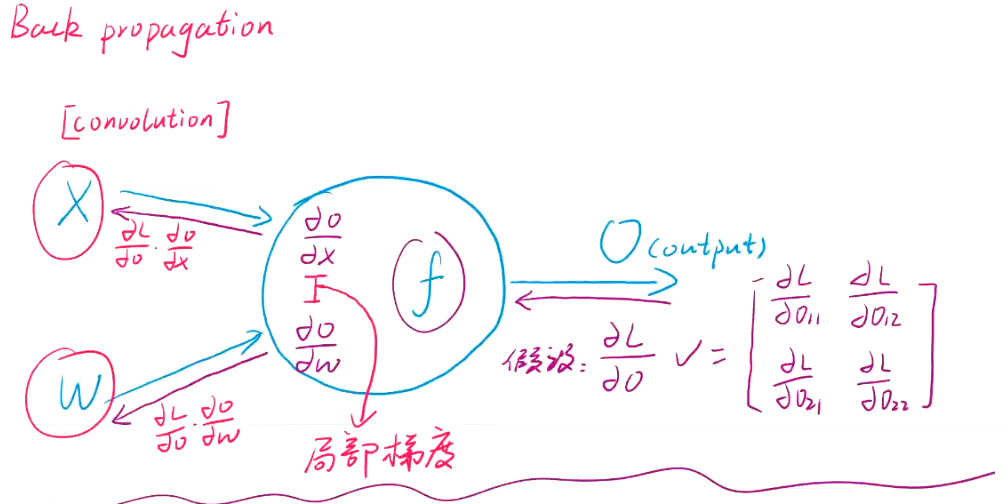这部分是由后面传过来的，是已知的。J表示损失函数。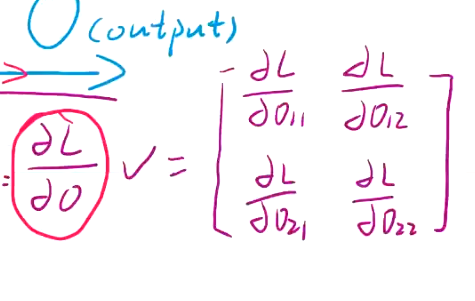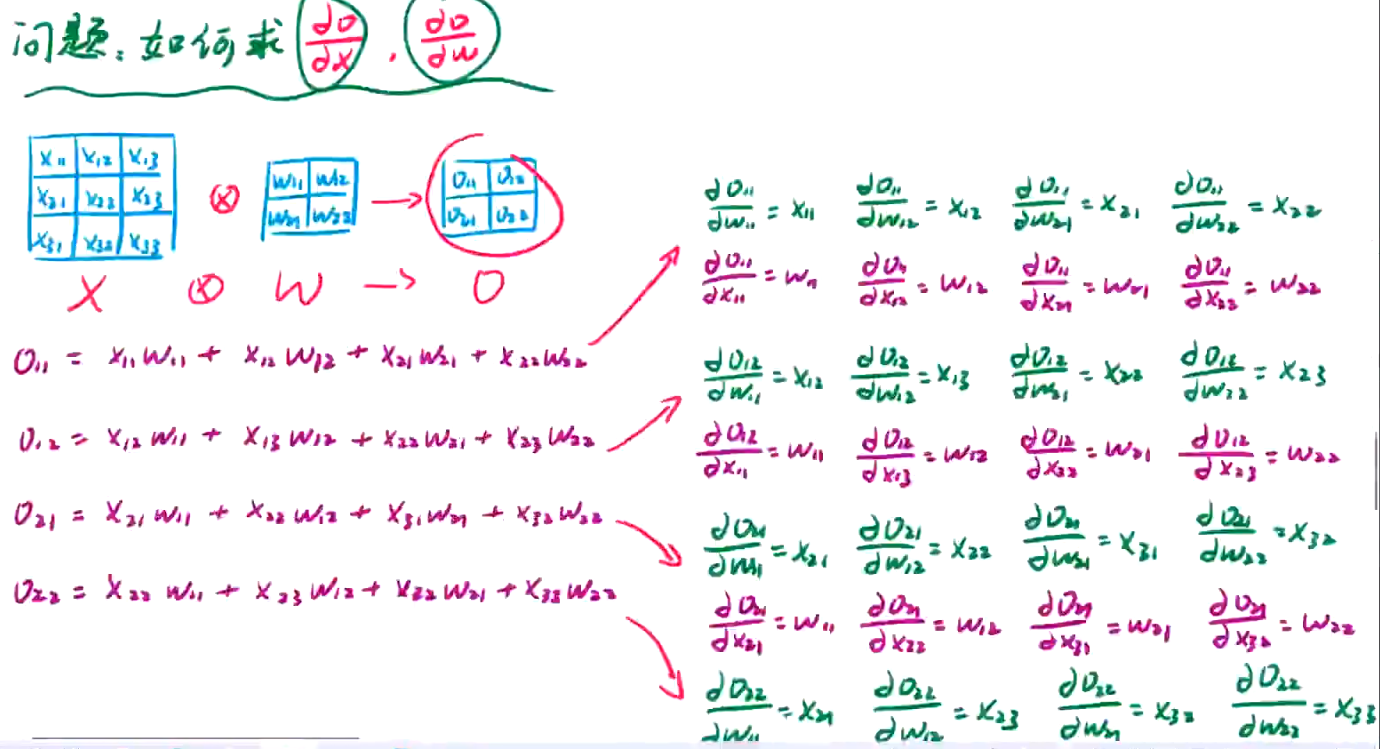接着求J对w的偏导；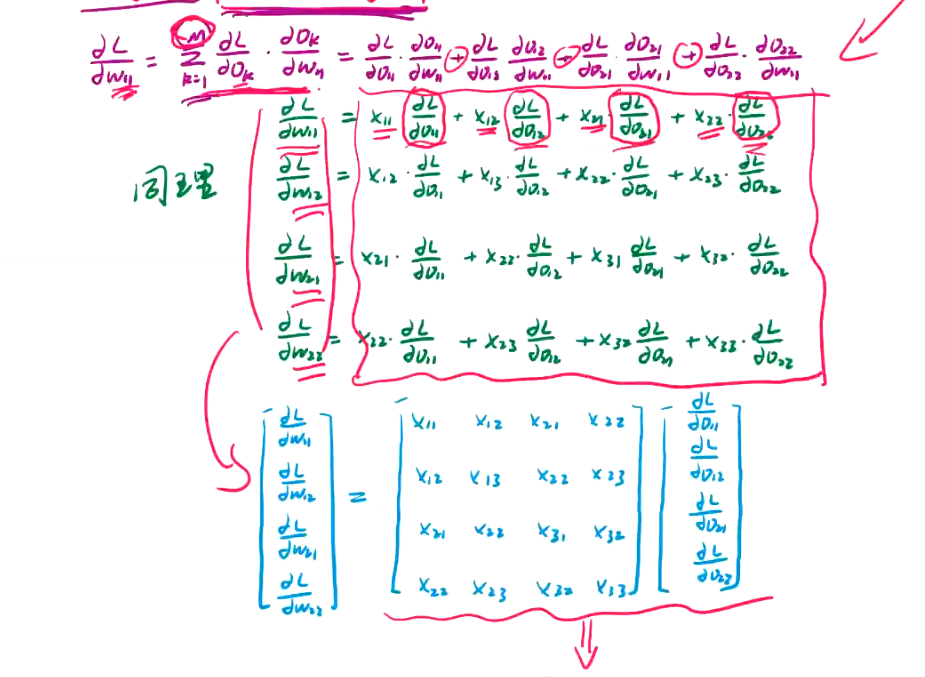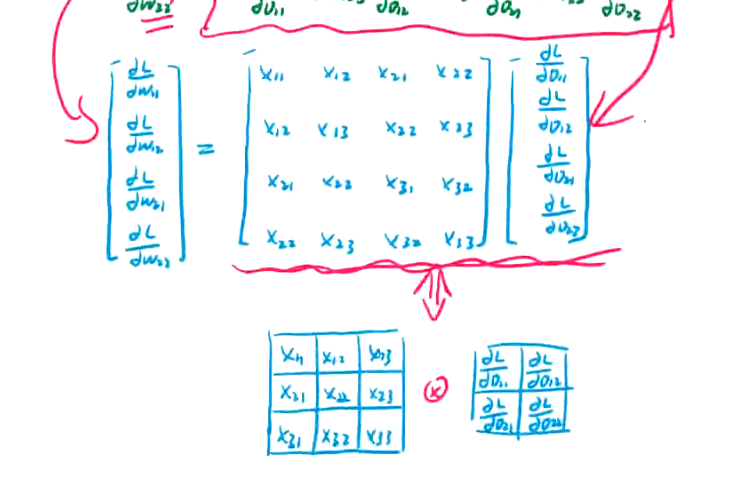接下来J对x求偏导：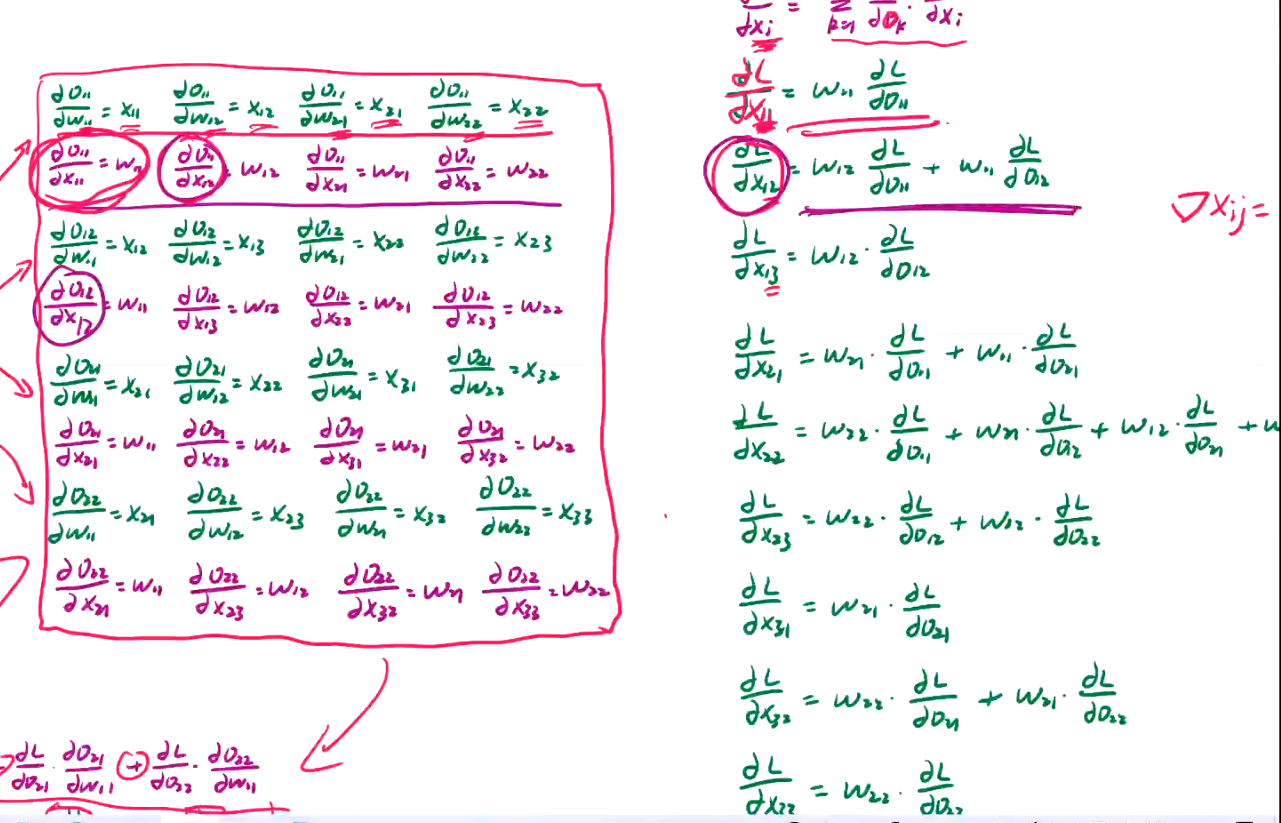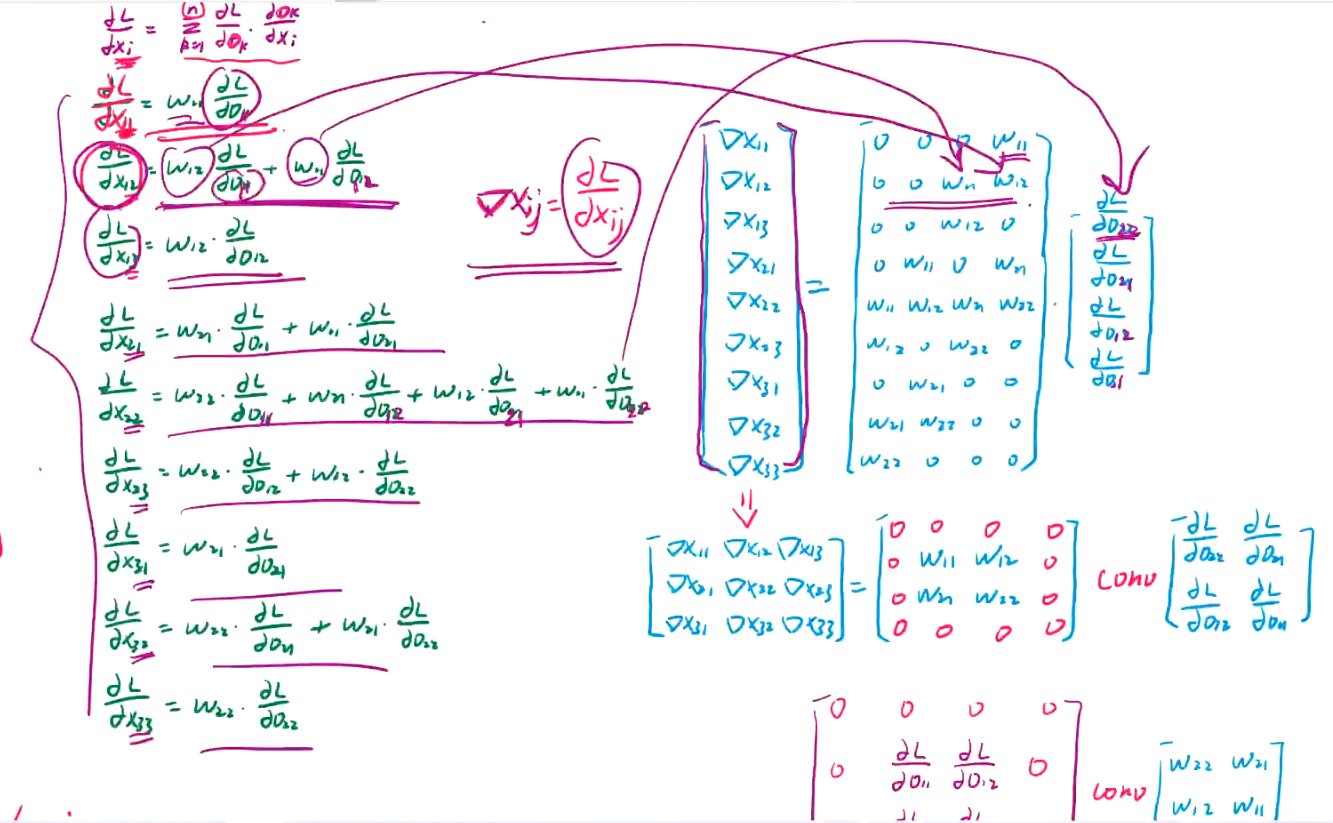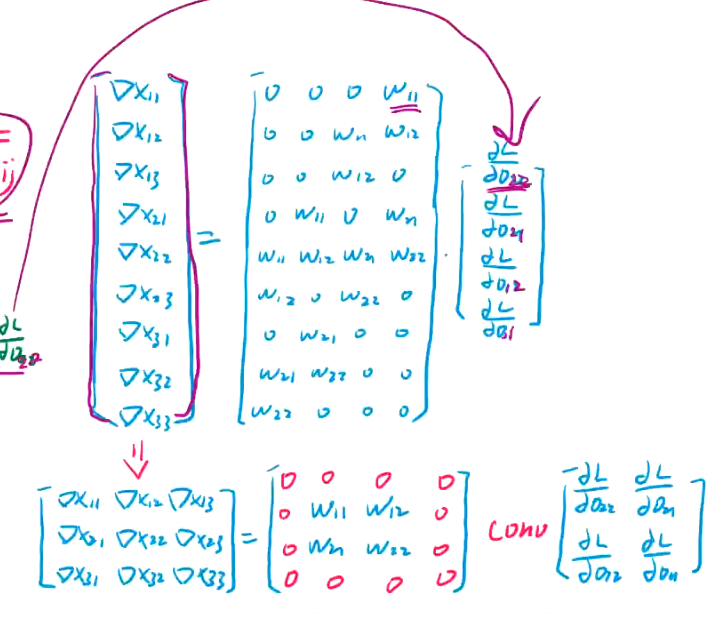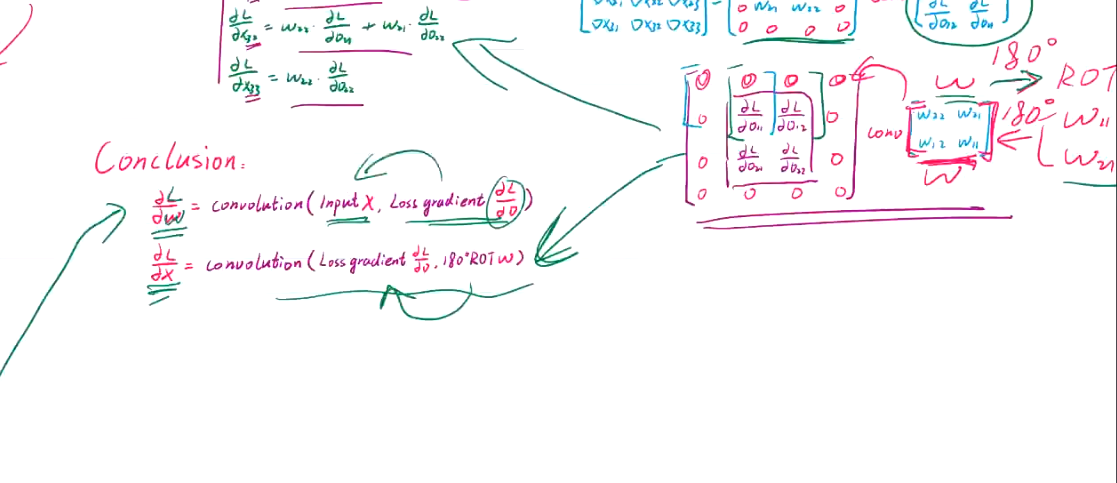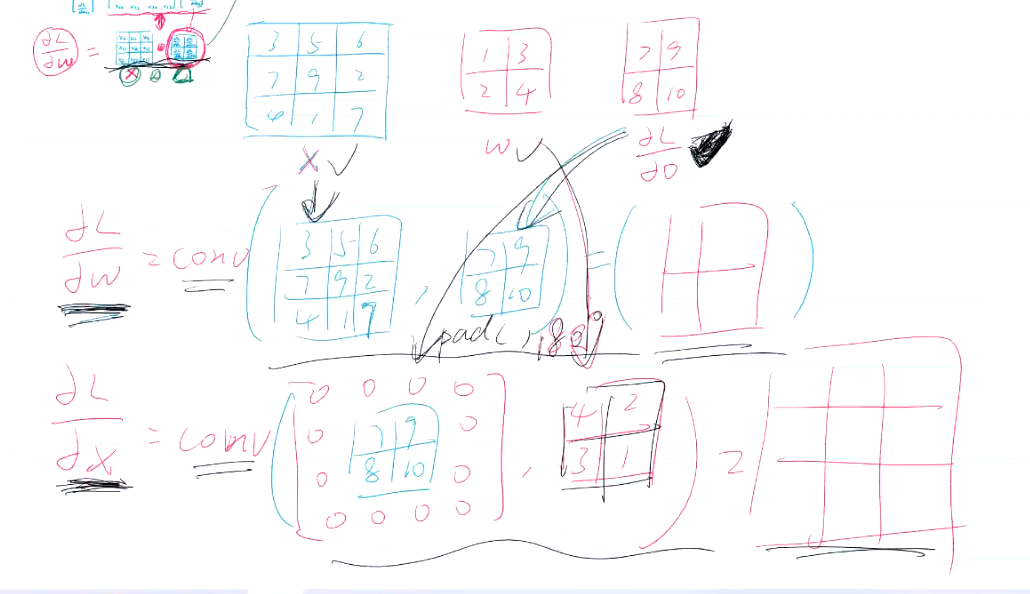b的梯度也是可以求出来的，就是上层传过来的梯度矩阵的所有元素和。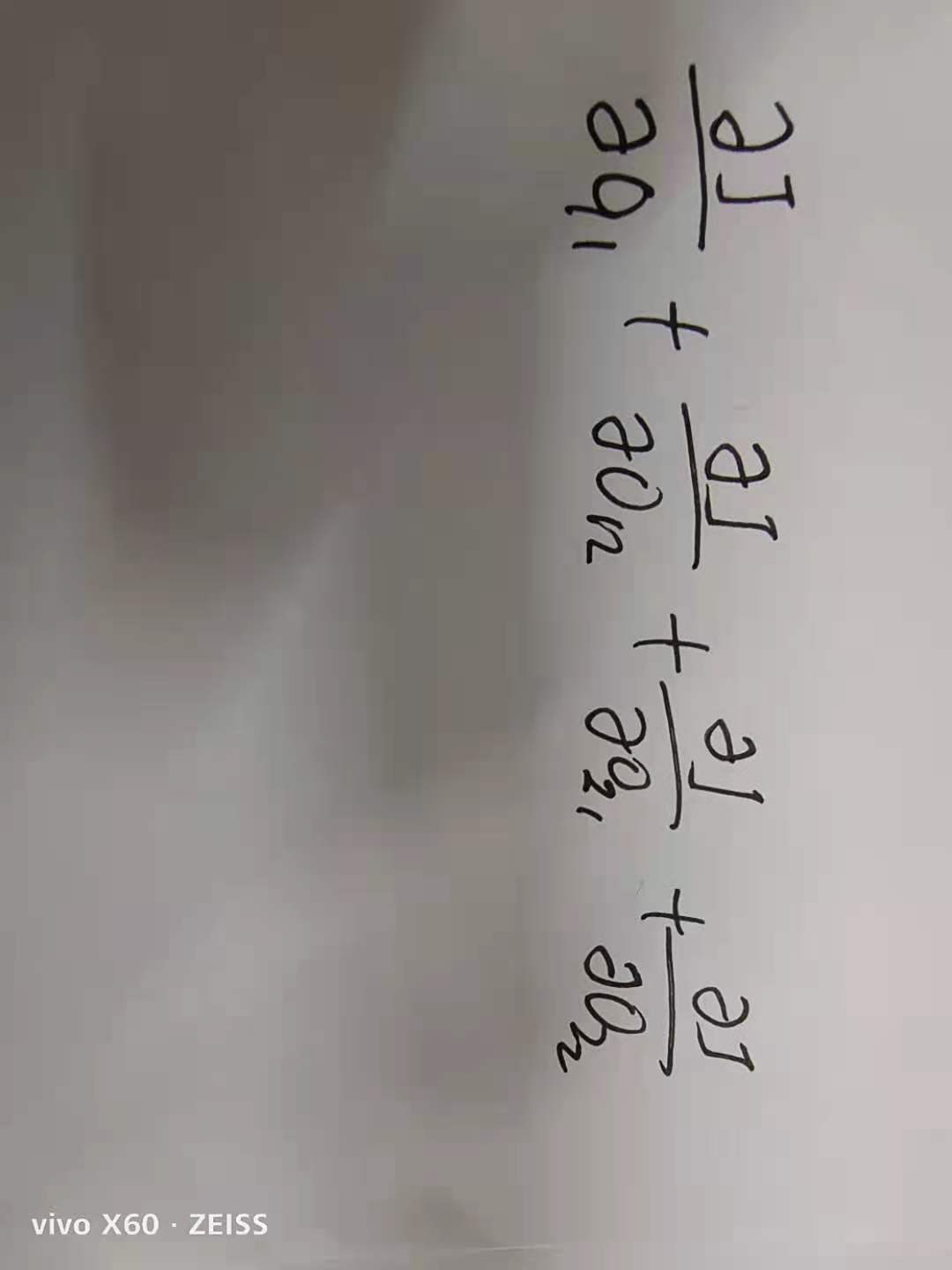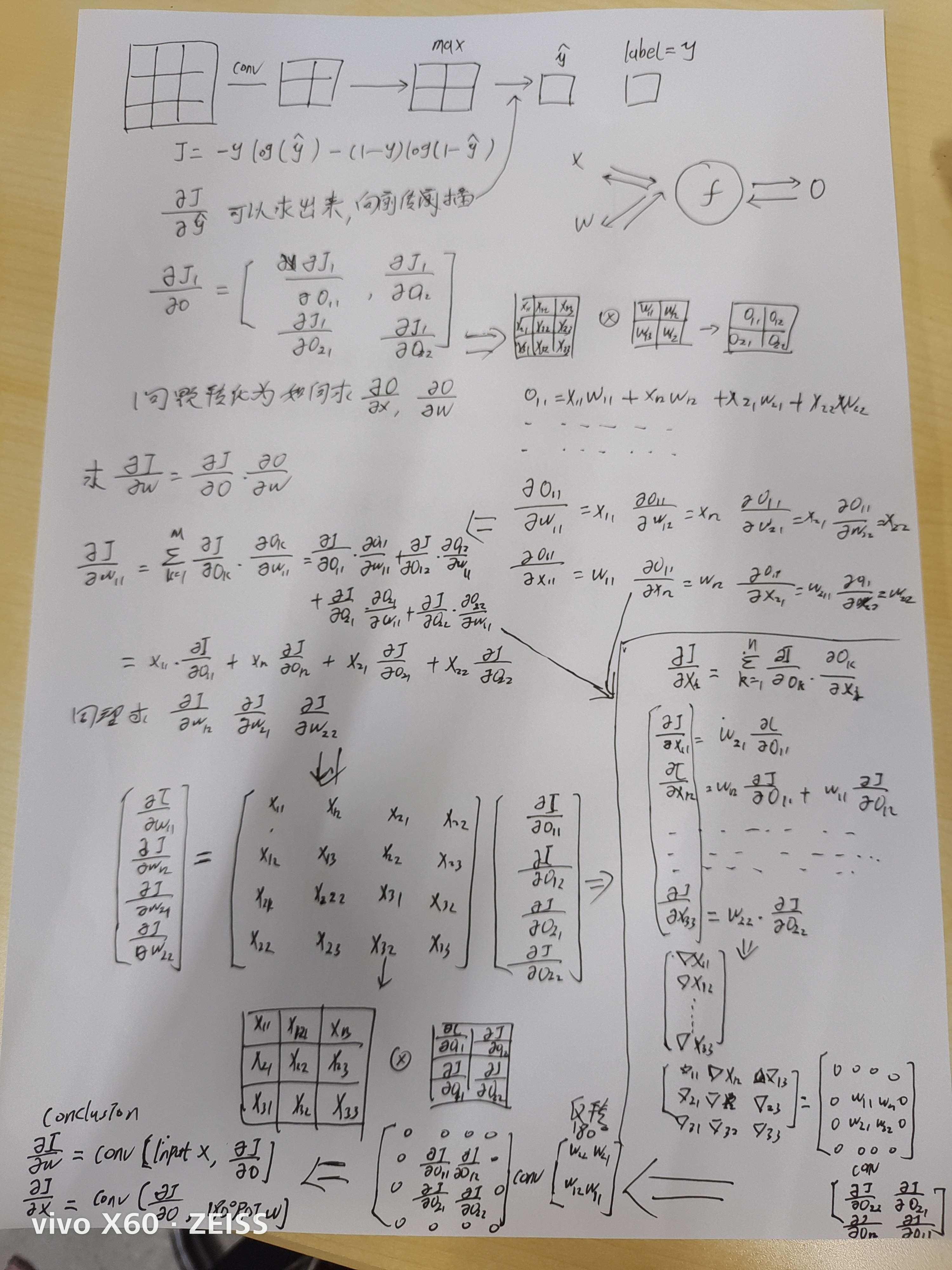展开全文深度学习 神经网络
• 我的学习笔记.参考：... ... 2.tensorflow中有求梯度的函数直接调用。可以自行验证。 3.代码： import pandas as pd import numpy as np import tensorflow as tf np.random.seed(0) def cros.

2.tensorflow中有求梯度的函数直接调用。可以自行验证。

3.代码：

import pandas as pd
import numpy as np
import tensorflow as tf
np.random.seed(0)

def crossentropy(y_pred,y_true):
return -tf.reduce_sum(y_true*tf.math.log(y_pred))

#---------input----------
x = tf.constant(np.random.randn(1, 9, 9, 1).astype(np.float32))
y_true = np.array([0.3,0.5,0.2]).astype(np.float32)
t.watch(x)                                #例子中的watch函数把需要计算梯度的变量x加进来了

#-----------conv l1---------------
l1 = tf.keras.layers.Conv2D(filters=1,kernel_size=(3,3),strides=2)
z_l1 = l1(x)
t.watch(z_l1)
a_l1 = tf.nn.relu(z_l1)
t.watch(a_l1)

#---------max pooling l2----------
l2 =  tf.keras.layers.MaxPool2D(pool_size=(2,2))
z_l2 = l2(a_l1)
t.watch(z_l2)
a_l2 = tf.keras.layers.Flatten()(z_l2) #3维转换成一维
t.watch(a_l2)

#--------------FNN L3----------------
l3 = tf.keras.layers.Dense(3)
z_l3 = l3(a_l2)
t.watch(z_l3)
a_l3 = tf.math.softmax(z_l3)
t.watch(a_l3)

#--------------loss----------------
loss = crossentropy(y_pred = a_l3,y_true = y_true)

print(z_l1)

print(l1.kernel.numpy())

print(a_l1)

展开全文tensorflow 深度学习 pytorch 神经网络
• 这也就说明了为什么我们需要千方百计的梯度！我们需要到达山底，就需要在每一步观测到此时最陡峭的地方，梯度就恰巧告诉了我们这个方向。梯度的方向是函数在给定点上升最快的方向，那么梯度的反方向就是函数在给...

关于梯度的概念

梯度是微积分中一个很重要的概念，之前提到过梯度的意义

• 在单变量的函数中，梯度其实就是函数的微分，代表着函数在某个给定点的切线的斜率
• 在多变量函数中，梯度是一个向量，向量有方向，梯度的方向就指出了函数在给定点的上升最快的方向。

这也就说明了为什么我们需要千方百计的求取梯度！我们需要到达山底，就需要在每一步观测到此时最陡峭的地方，梯度就恰巧告诉了我们这个方向。梯度的方向是函数在给定点上升最快的方向，那么梯度的反方向就是函数在给定点下降最快的方向，这正是我们所需要的。所以我们只要沿着梯度的方向一直走，就能走到局部的最低点！

2.3.2 梯度要乘以一个负号
梯度前加一个负号，就意味着朝着梯度相反的方向前进！我们在前文提到，梯度的方向实际就是函数在此点上升最快的方向！而我们需要朝着下降最快的方向走，自然就是负的梯度的方向，所以此处需要加上负号；那么如果时上坡，也就是梯度上升算法，当然就不需要添加负号了。
原文链接：https://blog.csdn.net/qq_41800366/article/details/86583789

梯度的更新

各种优化算法，其实就是对学习率的改变，有的是对学习率和上一次的梯度等综合考虑，来改变这一次的梯度的计算方法。

2.公式推导

我看的这篇推到文章，例题的形式很清晰

展开全文• 反向传播算法 本文是根据深度学习课程图文做的笔记 ...反向传播算法是一个有效求解梯度的算法，本质是链式求导法则的应用 1. 链式法则 利用链式求导法则：令 q=x+y 它们的微分： 又因为 q = x +y 则： ..

反向传播算法

本文是根据深度学习课程图文做的笔记

0. 背景

• 简单深度学习模型的基本流程：

1. 定义模型
2. 读入数据
3. 给出损失函数f
4. 梯度下降法更新参数（手动求梯度、pytorch自动求导）

对于复杂模型，如100层网络，该怎么解决呢？
⭐反向传播算法

• 自动求导的本质就是反向传播算法
• 反向传播算法是一个有效求解梯度的算法，本质是链式求导法则的应用

1. 链式法则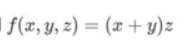利用链式求导法则：令 q=x+y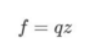它们的微分：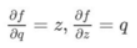又因为 q = x +y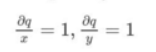则：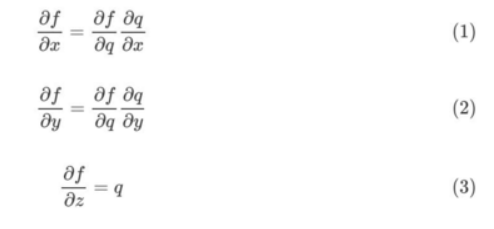链式法则/反向传播算法的核心：
如果需要对式子中元素求导，可以一层一层的求导然后将结果相乘

2. 反向传播算法

反向传播算法是链式法则的应用
q = x + y f = qz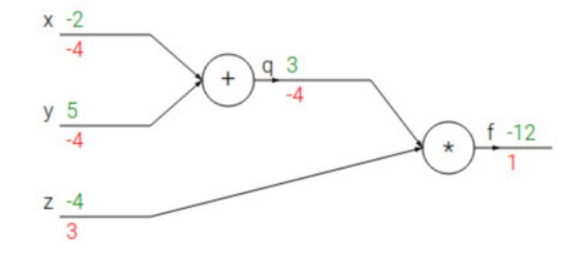绿色：数值 红色：求出的梯度
从最后开始的梯度是1 ：从后往前
f 对 q 和z 进行求导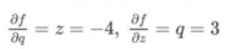接下来利用链式法则，q对x和y求导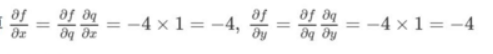反向传播算法：每次求导只对当前的运算求导
求解每层网络的参数都是用链式法则将前面的结果输出迭代到这一层，即一个传播的过程

3. Sigmoid函数示例反向传播的过程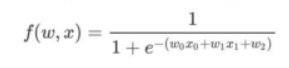需要求解：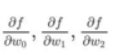从高到低，抽出函数的层数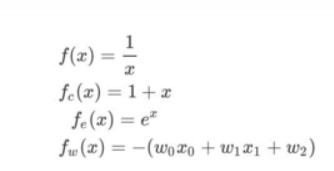计算图为: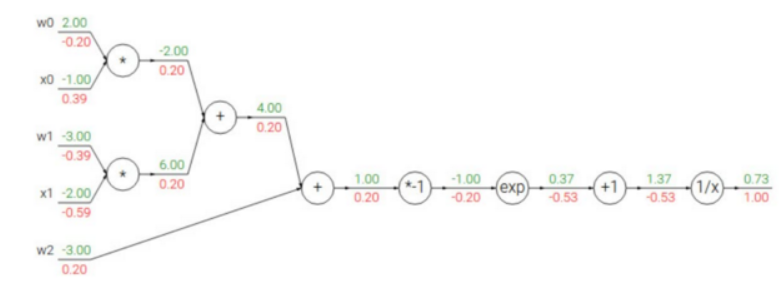绿色：数值 红色：梯度
从后往前计算每个参数的梯度
最后面的梯度是 1

1 / x 的 梯 度 是 − 1 / x 2 1/x 的梯度是 -1/x^{2}

1 ∗ ( − 1 ) / ( 1.37 ) 2 = − 0.53 1 * (-1)/(1.37)^{2} = -0.53

1 ∗ ( − 1 ) / ( 1.37 ) 2 = − 0.53 1 * (-1)/(1.37)^{2} = -0.53

+1 梯度不变

− 0.53 ∗ e − 1 = − 0.2 -0.53 * e^{-1} = -0.2
计算的时候注意x的数值，代入的时候要小心

按照这样的过程，不断往后传播，求得每个参数的梯度

展开全文算法 深度学习 网络 人工智能
• 定义一个简单的网络： 首先回顾前向过程，是这样的： ...开始推导反向传播了： 目标损失函数： 在本例当中就是： 我们以更新W4为例子来展开反向推导： 梯度更新W4 ： 根据链式法则： ...
• 这是一个刚学完反向传播的初学者很容易踩到的陷阱：试图不设中间变量，直接就把目标参数的梯度出来。如果这么去做的话，很容易在中间碰到这种非矩阵的结构，因为理论上矩阵对矩阵求导出来是一个4维tensor，...人工智能 神经网络 深度学习 机器学习 编程语言pytorch
• 计算图和反向传播 计算图：通过图的方式来描述函数的图形 J(a,b,c)=3(a+bc)J(a,b,c)=3(a+bc)J(a,b,c)=3(a+bc)，令u=a+v，v=bcu=a+v，v=bcu=a+v，v=bc，把它绘制成计算图可以表示为： 之后对每个节点偏导有： ...神经网络
• 激活函数先进行0-1区间，再输入损失函数与label进行误差计算，最后反向传播 常用交叉熵的原因 A)、原因在于交叉熵函数配合输出层的激活函数如sigmoid或softmax函数能更快地加速深度学习的训练速度 B)、因为反向传播...python airflow r语言
• import torch as t from torch.autograd import Variable as V ...def gradf(x): # 手动计算梯度 dx = 2 * x * t.exp(x) + x ** 2 * t.exp(x) return dx x = V(t.randn(3, 4), requires_grad=True) y = f(x
• 然后后面计算的所有新的梯度值都是通过对应的导数值与前一步计算的梯度值之间的乘积。 -0.53。由于f(x)=1/x的导数是-1/x2，所以-0.53=(-1/1.372)*1.00 -0.53。由于f(x)=c+x的导数是1，所以-0.53=1*(-0.53) -0.20。...深度学习 神经网络 数据分析 人工智能
• 一：反向传播 目标：给出输入数据i1,i2(0.05和0.10)，使输出尽可能与原始输出o1,o2(0.01和0.99)接近。 二：梯度下降 三：学习率
• 知道什么是反向传播 1. 梯度是什么？ 梯度：是一个向量，导数+变化最快的方向（学习的前进方向） 回顾机器学习 收集数据x，构建机器学习模型f，得到 f(x,w) = Ypredict 判断模型好坏的方法： 回归损失：loss = ...
• 神经网络与梯度下降，反向传播总结 引自3Blue1Brown的视频，b站上有它的官方账号。它的线性代数的原理，微积分的原理及神经网络系列都是神作 MLP多层感知机 3Blue1Brown ...一、MLP神经网络的结构和原理 ...计算机视觉 深度学习 机器学习
• 一个快速计算的方法就是反向传播。 该篇着重介绍这两个概念，偏数学一些，数学兴趣不浓的话可以快速浏览，懂不懂没关系，不影响后续学习。在实践中也不需要你懂得反向传播，函数库底层都封装好了，你直接调用即可。...神经网络 深度学习
• 梯度反向传播算法推导前言一、单个神经元的计算过程二、每层由单个神经元构成的神经网络三、每层由多个神经元构成的神经网络 前言 Backpropagation 反向传播算法推导过程 一、单个神经元的计算过程 output=σ...pytorch 深度学习 神经网络
• 上文(传送门)说到全连接神经网络的正向传播以及损失函数，通过损失函数值来评价网络的拟合效果，如何实现在训练过程中降低损失函数值呢？就涉及到本文的主要内容，反向传播以及梯度下降了。神经网络 深度学习
• 本质上是因为神经网络的更新方法，梯度消失是因为反向传播过程中对梯度的求解会产生sigmoid导数和参数的连乘，sigmoid导数的最大值为0.25，权重一般初始都在0，1之间，乘积小于1，多层的话就会有多个小于1的值连乘，...神经网络 深度学习
• 但对于多隐层的神经网络，输出层可以直接出误差来更新参数，但其中隐层的误差是不存在的，因此不能对它直接应用梯度下降，而是先将误差反向传播至隐层，然后再应用梯度下降，其中将误差从末层往前传递的过程需要...神经网络 机器学习
• 这篇文章讲一下RNN的反向传播算法BPTT，及RNN梯度消失和梯度爆炸的原因。 BPTT RNN的反向传播，也称为基于时间的反向传播算法BPTT(back propagation through time)。对所有参数损失函数的偏导，并不断调整这些...深度学习 人工智能 lstm
• 计算损失函数 optimizer.zero_grad() 手动清空过往梯度 loss.backward() 反向传播，根据loss值计算当前梯度 optimizer.step() 根据梯度更新网络参数 简单来说，就是进来一个batch的数据，计算一次梯度，更新一次网络...pytorch 深度学习
• 1.前向传播过程 ajl为第l层第j个神经元的...反向传播过程中要计算，我们先对代价函数做两个假设，以二次损失函数为例： 其中n为训练样本x的总数，y=y(x)为期望的输出，即ground truth，L为网络的层数，为...html5 机器学习 html
• 1.前向传播 前向传播算法：将上一层的输出作为下一层的输入，并计算下一层的输出，一直到运算到输出层为止。 对于Layer 2的输出 对于Layer 3的输出[公式]， [公式] [公式] 从上面可以看出，使用代数法一个个的...
• 梯度下降和反向传播1. 梯度是什么?2. 偏导的计算2.1 常见的导数计算2.2 多元函数偏导3. 反向传播算法3.1 计算图和反向传播3.2 神经网络中的反向传播3.2.1 神经网络的示意图3.2.2 神经网络的计算图 1. 梯度是什么? ...神经网络 深度学习 人工智能
• Python深度学习：梯度下降与反向传播 1、梯度 向量、学习（参数更新）方向 2、梯度下降 a、算出梯度 b、▽w = (f(w + Δx) - f(w - Δx)) / (2 * Δx) c、w = w - α▽w 3、常见的导数计算 导数的微分形式：牛顿...
• 首先要明白，误差回传与否，与 ...而权重和偏置值并不影响误差反向传播，误差反向传播计算的是每一层激活前神经元的偏导值。 计算激活前神经元偏导值DZ并不需要 DW 和 DB 的参与（一般涉及到DA 和 W）。 综上： p...神经网络
• 关于Pytorch中tensor的自动梯度求导与反向传播的理解 参考资料： 详解Pytorch 自动微分里的（vector-Jacobian product）； PyTorch 的 backward 为什么有一个 grad_variables 参数？； 【one way的pytorch学习笔记...python 深度学习 pytorch
• Pytorch梯度反向传播相关概念导数偏导数方向导数梯度梯度下降法自动求梯度概念Tensor反向传播求梯度 相关概念 先来理解一下从导数到梯度的相关概念。 导数 一元函数中导数就是该函数所代表的曲线在这一点上的切线...
• 反向传播算法（另梯度下降算法）定义算法推导一个具体的前向传播和反向传播算法的例子mse (均方误差）另两个问题梯度下降算法梯度数学解释梯度要乘以一个负号单变量函数的梯度下降多变量函数的梯度下降反向传播具体......